# Maharashtra Board 11th Commerce Maths Solutions Chapter 3 Complex Numbers Ex 3.1

Balbharati Maharashtra State Board 11th Commerce Maths Solution Book Pdf Chapter 3 Complex Numbers Ex 3.1 Questions and Answers.

## Maharashtra State Board 11th Commerce Maths Solutions Chapter 3 Complex Numbers Ex 3.1

Question 1.
Write the conjugates of the following complex numbers:
(i) 3 + i
(ii) 3 – i
(iii) -√5 – √7i
(iv) -√-5
(v) 5i
(vi) √5 – i
(vii) √2 + √3i
Solution:
(i) Conjugate of (3 + i) is (3 – i)
(ii) Conjugate of (3 – i) is (3 + i)
(iii) Conjugate of (-√5 – √7i) is (-√5 + √7i)
(iv) -√-5 = -√5 × √-1 = -√5i
Conjugate of -√-5 is √5i
(v) Conjugate of 5i is -5i
(vi) Conjugate of √5 – i is √5 + i
(vii) Conjugate of √2 + √3i is √2 – √3i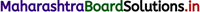Question 2.
Express the following in the form of a + ib, a, b ∈ R, i = √-1. State the values of a and b:
(i) (1 + 2i)(-2 + i)
(ii) $$\frac{\mathrm{i}(4+3 \mathrm{i})}{(1-\mathrm{i})}$$
(iii) $$\frac{(2+i)}{(3-i)(1+2 i)}$$
(iv) $$\frac{3+2 i}{2-5 i}+\frac{3-2 i}{2+5 i}$$
(v) $$\frac{2+\sqrt{-3}}{4+\sqrt{-3}}$$
(vi) (2 + 3i)(2 – 3i)
(vii) $$\frac{4 i^{8}-3 i^{9}+3}{3 i^{11}-4 i^{10}-2}$$
Solution: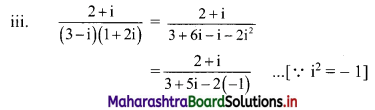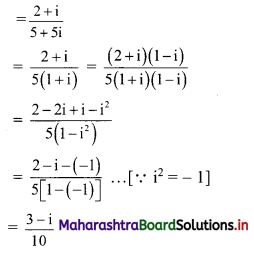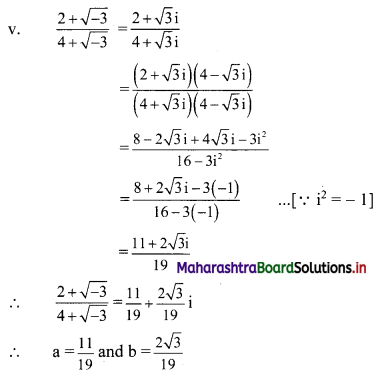Question 3.
Show that (-1 + √3i)3 is a real number.
Solution:
(-1 + √3i)3
= (-1)3 + 3(-1)2 (√3i) + 3(-1)(√3i)2 +(√3i)3 [∵ (a + b)3 = a3 + 3a2b + 3ab2 + b3]
= -1 + 3√3i – 3(3i2) + 3√3 i3
= -1 + 3√3i – 3(-3) – 3√3i [∵ i2 = -1, i3 = -1]
= -1 + 9
= 8, which is a real number.

Question 4.
Evaluate the following:
(i) i35
(ii) i888
(iii) i93
(iv) i116
(v) i403
(vi) $$\frac{1}{i^{58}}$$
(vii) i30 + i40 + i50 + i60
Solution:
We know that, i2 = -1, i3 = -i, i4 = 1
(i) i35 = (i4)8 (i2) i = (1)8 (-1) i = -i
(ii) i888 = (i4)222 = (1)222 = 1
(iii) i93 = (i4)23 . i = (1)23 . i = i
(iv) i116 = (i4)29 = (1)29 = 1
(v) i403 = (i4)100 (i2) i = (1)100 (-1) i = -i
(vi) $$\frac{1}{i^{88}}=\frac{1}{\left(i^{4}\right)^{14} \cdot i^{2}}=\frac{1}{(1)^{14}(-1)}=-1$$
(vii) i30 + i40 + i50 + i60
= (i4)7 i2 + (i4)10 + (i4)12 i2 + (i4)15
= (1)7 (-1) + (1)10 + (1)12 (-1) + (1)15
= -1 + 1 – 1 + 1
= 0Question 5.
Show that 1 + i10 + i20 + i30 is a real number.
Solution:
1 + i10 + i20 + i30
= 1 + (i4)2 . i2 + (i4)5 + (i4)7 . i2
= 1 + (1)2 (-1) + (1)5 + (1)7 (-1) [∵ i4 = 1, i2 = -1]
= 1 – 1 + 1 – 1
= 0, which is a real number.

Question 6.
Find the value of
(i) i49 + i68 + i89 + i110
(ii) i + i2 + i3 + i4
Solution:
(i) i49 + i68 + i89 + i110
= (i4)12 . i + (i4)17 + (i4)22 . i + (i4)27 . i2
= (1)12 . i + (1)17 + (1)22 . i + (1)27(-1) ……[∵ i4 = 1, i2 = -1]
= i + 1 + i – 1
= 2i

(ii) i + i2 + i3 + i4
= i + i2 + i2 . i + i4
= i – 1 – i + 1 [∵ i2 = -1, i4 = 1]
= 0

Question 7.
Find the value of 1 + i2 + i4 + i6 + i8 + …… + i20.
Solution:
1 + i2 + i4 + i6 + i8 + ….. + i20
= 1 + (i2 + i4) + (i6 + i8) + (i10 + i12) + (i14 + i16) + (i18 + i20)
= 1 + [i2 + (i2)2] + [(i2)3 + (i2)4] + [(i2)5 + (i2)6] + [(i2)7 + (i2)8] + [(i2)9 + (i2)10]
= 1 + [-1 + (- 1)2] + [(-1)3 + (-1)4] + [(-1)5 + (-1)6] + [(-1)7 + (-1)8] + [(-1)9 + (-1)10] [∵ i2 = -1]
= 1 + (-1 + 1) + (-1 + 1) + (-1 + 1) + (-1 + 1) + (-1 + 1)
= 1 + 0 + 0 + 0 + 0 + 0
= 1

Question 8.
Find the values of x and y which satisfy the following equations (x, y ∈ R):
(i) (x + 2y) + (2x – 3y)i + 4i = 5
(ii) $$\frac{x+1}{1+\mathrm{i}}+\frac{y-1}{1-\mathrm{i}}=\mathrm{i}$$
Solution:
(i) (x + 2y) + (2x – 3y)i + 4i = 5
∴ (x + 2y) + (2x – 3y)i = 5 – 4i
Equating real and imaginary parts, we get
x + 2y = 5 ……..(i)
and 2x – 3y = -4 ………(ii)
Equation (i) × 2 – equation (ii) gives
7y = 14
∴ y = 2
Putting y- 2 in (i), we get
x + 2(2) = 5
∴ x + 4 = 5
∴ x = 1
∴ x = 1 and y = 2
Check:
If x = 1 and y = 2 satisfy the given condition, then our answer is correct.
L.H.S. = (x + 2y) + (2x – 3y)i + 4i
= (1 + 4) + (2 – 6)i + 4i
= 5 – 4i + 4i
= 5
= R.H.S.

(ii) $$\frac{x+1}{1+\mathrm{i}}+\frac{y-1}{1-\mathrm{i}}=\mathrm{i}$$(x + y) + (y – x – 2)i = 2i
(x + y) + (y – x – 2)i = 0 + 2i
Equating real and imaginary parts, we get
x + y = 0 and y – x – 2 = 2
∴ x + y = 0 ……(i)
and -x + y = 4 ……..(ii)
Adding (i) and (ii), we get
2y = 4
∴ y = 2
Putting y = 2 in (i), we get
x + 2 = 0
∴ x = -2
∴ x = -2 and y = 2Question 9.
Find the value of:
(i) x3 – x2 + x + 46, if x = 2 + 3i
(ii) 2x3 – 11x2 + 44x + 27, if x = $$\frac{25}{3-4 i}$$
Solution:
(i) x = 2 + 3i
∴ x – 2 = 3i
∴ (x – 2)2 = 9i2
∴ x2 – 4x + 4 = 9(-1) …..[∵ i2 = -1]
∴ x2 – 4x + 13 = 0 ……(i)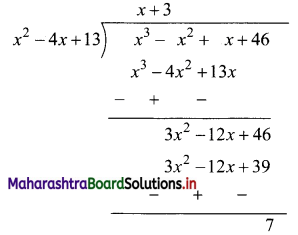∴ x3 – x2 + x + 46 = (x2 – 4x + 13)(x + 3) + 7
= 0(x + 3) + 7 ……[From (i)]
= 7

(ii) x = $$\frac{25}{3-4 i}$$∴ x = 3 + 4i
∴ x – 3 = 4i
∴ (x – 3)2 = 16i2
∴ x2 – 6x + 9 = 16(-1) …….[∵ i2 = -1]
∴ x2 – 6x + 25 = 0 …….(i)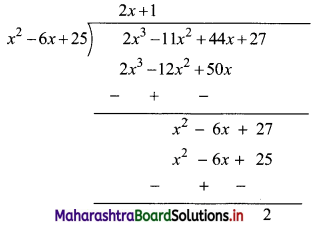∴ 2x3 – 11x2 + 44x + 27
= (x2 – 6x + 25) (2x + 1) + 2
= 0 . (2x + 1) + 2 ……[From (i)]
= 0 + 2
= 2